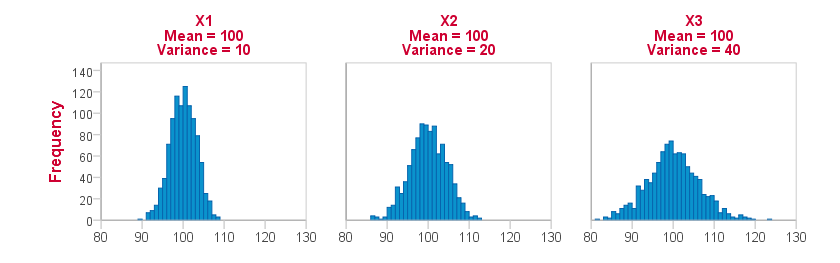SPSS TUTORIALS BASICS ANOVA REGRESSION FACTOR CORRELATION

# Descriptive Statistics – One Metric Variable

## Introduction

A previous tutorial introduced some summary statistics appropriate for both categorical as well as metric variables. Now it's time to turn to some measures that apply to metric variables exclusively. The most important ones are the mean (or average), variance and standard deviation.

## Mean

Most of us are probably familiar with the mean (or average) but we'll briefly review it for the sake of completeness. The mean is the sum of all values divided by the number of values that were added. We can represent this definition by the formula $$\overline{X} = \frac{\sum\limits_{i=1}^n X_i}{n}$$ in which

• $$\overline{X}$$ is the mean for variable $$X$$;
• $$\sum\limits_{i=1}^n$$ means that we sum over all values;
• $$X_i$$ is a value from $$X$$ and;
• $$n$$ is the number of values that we're adding.

## Example Calculation Mean

Now let's say we have some variable X1 containing the values 8, 9, 10, 11 and 12. If we fill these out in our formula, we'll see that the mean of these values is 10: $$\overline{X} = \frac{8 + 9 + 10 + 11 +12}{5} = 10$$

## Variance

The variance is the average squared deviation from the mean. We can represent this definition by the formula $$S^2 = \frac{\sum\limits_{i=1}^n(X_i - \overline{X})^2}{n}$$
The variance is a measure of dispersion; it indicates how far the data values lie apart.

## Example Calculation Variance

Let's reconsider variable X1 holding values 8, 9, 10, 11 and 12. If we apply the formula, we'll find that the variance is 2.Statistical software may come up with the value 2.5 here. This is because it divides the sum by (n - 1) instead of n. The difference between the two approaches is beyond the scope of this tutorial but we'll explain it in due time. $$S^2 = \frac{(8-10)^2 + (9-10)^2 + (...) + (12-10)^2}{5} = 2$$
Now we have a second variable, X2, holding values 6, 8, 10, 12 and 14. How would you describe in words the difference between variables X1 and X2? They both have a mean value of 10. Well, the difference is that the values of X2 lie further apart; that is, X2 has a larger variance than X1.

## Variance and Histogram

A variable's variance is reflected by the shape of its histogram. Everything else equal, as the variance increases, the histogram becomes wider and lower. The figure below illustrates this for real data. Each variable has 1,000 observations and a mean of precisely 100. Note that the three histograms use the same scales for their horizontal and vertical axes.Note how the histograms become lower and wider as variance increases.

## Standard Deviation

The standard deviation is the square root of the variance. Its formula is therefore almost identical to that of the variance: $$S = \sqrt{\frac{\sum_{i=1}^n(X_i - \overline{X})^2}{n}}$$
Just like the variance, the standard deviation is a measure of dispersion; it indicates how far a number of values lie apart.

The standard deviation and the variance thus basically express the same thing, albeit on different scales. So why don't we just use one measure for expression the dispersion of a number of values? The reason is that for some scenarios the standard deviation is mathematically more convenient and reversely for the variance.

# Tell us what you think!

*Required field. Your comment will show up after approval from a moderator.

# THIS TUTORIAL HAS 5 COMMENTS:

•### By mahros darsin on November 2nd, 2016

Easy to understand!

•### By Aregawi Abrha on October 15th, 2017

I really appreciate the coarse it so vital for me to know and to have a deep knowledge of this statically software further I need your more help in such courses .and thank you for your positive efforts.

•### By Yonah on November 14th, 2017

Wish to discover how much I dont know

•### By Lloyd on July 13th, 2018

Easy step by step explanation.

•